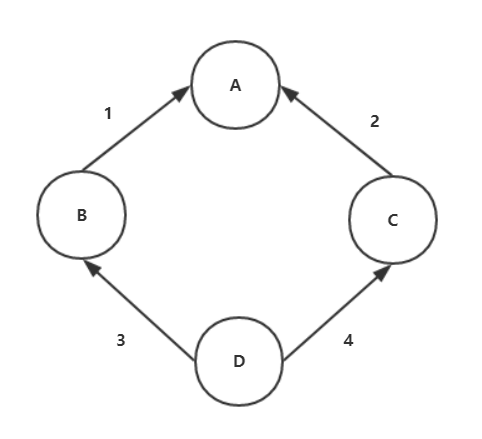## python 面向对象之继承顺序

先来看一个经典类

```class A:
def __init__(self):
print('A')
class B(A):
def __init__(self):
print('B')
class C(A):
def __init__(self):
print('C')
class D(B,C):
pass

obj = D()```

继承顺序如下图：B如果有构造函数，就不会继承C的构造函数

找到第一个构造函数，就停下来，不再寻找了。

构造函数只会执行一次，先从本身找，找不到，就去上层寻找，顺序如下：

D->B->C->A

执行上面的代码，输出：B

为什么会输出B呢？往下看

将B的构造函数注释掉

```class A:
def __init__(self):
print('A')
class B(A):
pass
# def __init__(self):
#     print('B')
class C(A):
def __init__(self):
print('C')
class D(B,C):
pass

obj = D()```

执行输出：C

将C的构造函数注释掉

```class A:
def __init__(self):
print('A')
class B(A):
pass
# def __init__(self):
#     print('B')
class C(A):
pass
# def __init__(self):
#     print('C')
class D(B,C):
pass

obj = D()```

执行输出：A

这是一个正常的寻找顺序

那么问题来了

B虽然没有构造函数，但是它继承了A，它应该从A中继续寻找构造函数才对啊

为什么去找C呢？

因为D找B的时候，B发现没有，虽然B可以从A中继承，A是更高层的，先不找它。

BC是属于同一层的(继承B,C)，既然B没有，那么去找C了。

这种查询策略，叫做广度优先

先从横向策略(D->B->C->A) 查找，如果找不到了，再从上层找。

查找有很多策略

上面说到B没有，不应该就这么结束了，直接去找C了。应该从A中查找，A如果没有，再找C，顺序如下：

D->B->A->C

这种查询策略，叫做深度优先

这里没有所谓的对错，是2种查询策略，也叫继承策略

在不同的场景下，会选择不同的查询策略

从上面的例子可以看出，是属于广度优先

从python3开始，都是广度优先

使用python2执行如下代码：

```class A():
def __init__(self):
print('A')
class B(A):
pass
# def __init__(self):
#     print('B')
class C(A):
def __init__(self):
print('C')
class D(B,C):
pass

obj = D()```

执行输出：A

可以看出，python2使用的是 深度优先

上面的代码是经典类写法

下面看一下新式类的写法

只是将A()改为A(object)

```class A(object):
def __init__(self):
print('A')
class B(A):
pass
# def __init__(self):
#     print('B')
class C(A):
def __init__(self):
print('C')
class D(B,C):
pass

obj = D()```

使用python3和python2执行

结果都是C

总结：

python2 经典类是按深度优先来继承的，新式类是按广度优先来继承的

python3 经典类和新式类都是统一按广度优先来继承的

在python2中，继承效率来讲，深度优先不如广度优先

所以在python3中，统一改成广度优先